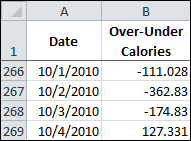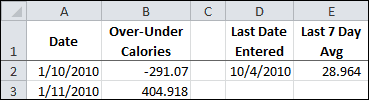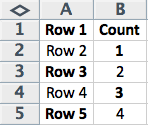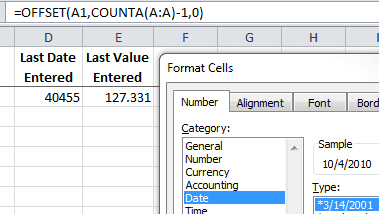# Become the OFFSET Function and Tell a Short Story

The OFFSET Function is a difficult one to learn. I’ve decided to become the OFFSET function and tell a simple story of how I give you a value for the last cell in a range by using an example.

The example data is a spreadsheet I use to log my over-under calories to goal each day. This data is downloaded periodically and currently the data goes down to row 269, as you can see below.I use the OFFSET Function at the top of the worksheet to inform me of the Last Date Entered in the Date column, as shown below.Cell D2 has the following formula to pick up the Last Date Entered from column A.

=OFFSET(A1,COUNTA(A:A)-1,0)

## I Am the OFFSET Function

The first thing you have to understand about me is that I will take the arguments you give and return a reference. And by that I mean a reference to a range. In this example I return a single cell reference, A269.

Three arguments are given in this example, which is good because they’re all required for me to give you an answer. There are two optional arguments that weren’t provided and I won’t worry about those now.

The first argument given is the cell reference A1. This is my reference point, or ground zero as I like to call it. Everything starts here. This range reference has two components, row 1 and column A, which are reference points for the following arguments.

The second argument is rows, which is a number I can use for the row offset. The formula COUNTA(A:A) – 1 is given for me to decipher.

The COUNTA function has the argument A:A, which is column A. This function will count all the non-blank cells in column A, which happens to be 269 for this example. Then by subtracting one, I get 268 rows for the second argument. Note: By using the COUNTA Function you assume there are no blank cells in the column A data. Important if you want the correct answer for the last data point.

Now that I have 268 for my row offset number in the second argument, I’ll just count down from the reference point, row 1. And the reference row is ground  zero in counting down. So row 2 is count 1, row 3 is count 2, row 4 is count 3, and so on until I reach 268. Here’s an example of how I’m counting with the row offset, starting with row 1.You’ll notice the count is always one less than the number of rows, which explains the formula in the second argument, COUNTA(A:A) – 1.

The third argument is cols, which is a number I’ll use for the column offset. Since I’ve been given a zero in this example I’ll stay in column A, which is the reference column.

Finally, after taking the reference cell A1 and moving down 268 rows and over zero columns I will return the range reference A269, which gives you the last data point in column A.

Surprise! Cell D2 shows the number 40455, which is the date serial number.It’s up to you to format cell D2 with a date format. My job is done.